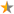40,241 Pages

StageStatusDescription
Template documentation (for the template shown above, sometimes hidden or invisible)

## Contents

Template for quest stages in Gamebryo-engine games (like Fallout 3).

## Usage

```{{Quest stage table
|stage1   =
|status1  =
|desc1    =
|stage2   =
|status2  =
|desc2    =
...
|stage50  =
|status50 =
|desc50   =
}}
```

### Parametersstage1 up to stage50
Quest stage ID in G.E.C.K. ("Quest" edit window, "Quest Stages" tab, "Index" column).status1 up to status50
Quest status change (if any). Leave empty if no status change.

Available options:

• fail: This stage marks the quest as failed.
• finish: This stage marks the quest as finished.desc1 up to desc50
Description of quest stage (i.e. explanation when this stage is set).

## Example

```{{Quest stage table
|stage1   =5
|status1  =
|desc1    =Player has been tasked to make a template.
|stage2   =10
|status2  =fail
|desc2    =The template is not working.
|stage3   =15
|status3  =finish
|desc3    =Player has finished the template.
}}
```

results in:

StageStatusDescription
5 Player has been tasked to make a template.
10The template is not working.
15Player has finished the template.

## Copy/paste

More than 30 will rarely be needed; expand if necessary.

```{{Quest stage table
|stage1   =
|status1  =
|desc1    =
|stage2   =
|status2  =
|desc2    =
|stage3   =
|status3  =
|desc3    =
|stage4   =
|status4  =
|desc4    =
|stage5   =
|status5  =
|desc5    =
|stage6   =
|status6  =
|desc6    =
|stage7   =
|status7  =
|desc7    =
|stage8   =
|status8  =
|desc8    =
|stage9   =
|status9  =
|desc9    =
|stage10  =
|status10 =
|desc10   =
|stage11  =
|status11 =
|desc11   =
|stage12  =
|status12 =
|desc12   =
|stage13  =
|status13 =
|desc13   =
|stage14  =
|status14 =
|desc14   =
|stage15  =
|status15 =
|desc15   =
|stage16  =
|status16 =
|desc16   =
|stage17  =
|status17 =
|desc17   =
|stage18  =
|status18 =
|desc18   =
|stage19  =
|status19 =
|desc19   =
|stage20  =
|status20 =
|desc20   =
|stage21  =
|status21 =
|desc21   =
|stage22  =
|status22 =
|desc22   =
|stage23  =
|status23 =
|desc23   =
|stage24  =
|status24 =
|desc24   =
|stage25  =
|status25 =
|desc25   =
|stage26  =
|status26 =
|desc26   =
|stage27  =
|status27 =
|desc27   =
|stage28  =
|status28 =
|desc28   =
|stage29  =
|status29 =
|desc29   =
|stage30  =
|status30 =
|desc30   =
}}
```
Documentation transcluded from Template:Quest stage table/doc.
Community content is available under CC-BY-SA unless otherwise noted.